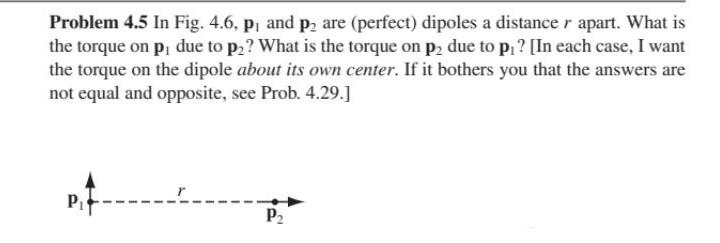# Question Could you please answer this question in full detail with all the directions explicit and explanations. Every time I give this question a try I get confused when it comes to directions since these are polar coordinates (for Edipole). If you could, do not use magnitudes to solve the question so I can understand why the torque is in the direction calculated. Problem 4.5 In Fig. 4.6, P, and p2 are (perfect) dipoles a distance r apart. What is the torque on p, due to p2? What is the torque on p2 due to p ? [In each case, I want the torque on the dipole about its own center. If it bothers you that the answers are not equal and opposite, see Prob. 4.29.] Pit P2